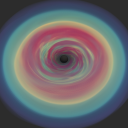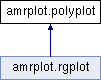BHAC Python tools
amrplot.polyplot Class Reference

Simple 2D plotter class using matplotlib, plots every cell in one patch. More...

Inheritance diagram for amrplot.polyplot:## Public Member Functions

def __init__ (self, value, data, nlevels=256, grid=None, blocks=None, blockWidth=8, blockHeight=8, nlevel1=0, cmap='jet', min=None, max=None, xrange=None, yrange=None, orientation='vertical', right=True, fixzoom=None, fixrange=None, fig=None, axis=None, filenameout=None, clear=True, edgecolor='k', smooth=0, swap=0, kwargs)

def setValue (self, value, min=None, max=None)
Sets the min and max values of the data to saturate the display. More...

def update (self, var=None, data=None, min=None, max=None, reset=None, fixrange=None, filenameout=None)
Prepare to re-draw the window, check if data was updated. More...

def info (self)
Print info to the console. More...

def show (self, var=None, data=None, min=None, max=None, reset=None, fixrange=None, filenameout=None)
Draw the plotting-window. More...

def colorbar (self, cax=None)
Draw the colorbar. More...

def save (self, filenameout=None)
Save the figure. More...

def onkey (self, event)

swap

nlevels

grid

blocks

blockWidth

blockHeight

nlevel1

cmap

orientation

right

cbarwidth

fixzoom

fixrange

filenameout

clear

fontsize

fig_w

fig_h

dpi

maxXticks

maxYticks

cbarticks

edgecolor

smooth

xrange

yrange

figure

ax

value

min

max

data

viewXrange

viewYrange

valueClip

xlist

ylist

xlistspecial

ylistspecial

cax

cbar

selection

## Detailed Description

Simple 2D plotter class using matplotlib, plots every cell in one patch.

Definition at line 26 of file amrplot.py.

## ◆ __init__()

 def amrplot.polyplot.__init__ ( self, value, data, nlevels = `256`, grid = `None`, blocks = `None`, blockWidth = `8`, blockHeight = `8`, nlevel1 = `0`, cmap = `'jet'`, min = `None`, max = `None`, xrange = `None`, yrange = `None`, orientation = `'vertical'`, right = `True`, fixzoom = `None`, fixrange = `None`, fig = `None`, axis = `None`, filenameout = `None`, clear = `True`, edgecolor = `'k'`, smooth = `0`, swap = `0`, kwargs )

Definition at line 33 of file amrplot.py.

33  swap=0,**kwargs):
34
35  self.swap=swap
36  self.nlevels=nlevels
37  self.grid = grid
38  self.blocks = blocks
39  self.blockWidth = blockWidth
40  self.blockHeight = blockHeight
41  self.nlevel1 = nlevel1
42  self.cmap=cmap
43  self.orientation=orientation
44  self.right=right # If True, colorbar is at the right, if False it is at the left
45  self.cbarwidth=0.15
47  self.fixzoom=fixzoom
48  self.fixrange=fixrange
49  self.filenameout=filenameout
50  self.clear=clear # If True, the figure is cleared when initializing amrplot, preventing us e.g. for drawing on a different subplot.
51
52  self.fontsize=10
53  self.fig_w=2.5
54  self.fig_h=4
55  self.dpi=300
56  self.maxXticks=None
57  self.maxYticks=None
58  self.cbarticks=None
59  self.edgecolor=edgecolor
60  self.smooth=smooth
61
62  self.xrange=xrange
63  self.yrange=yrange
64  if xrange==None:
65  self.xrange=[data.getBounds(),data.getBounds()]
66  if yrange==None:
67  self.yrange=[data.getBounds(),data.getBounds()]
68
69  self.setValue(value,min=min,max=max)
70
71  # initialize for screen:
72  # If a figure and axis were not given, create new ones
73  if fig==None:
74  self.figure=plt.figure(figsize=(self.fig_w,self.fig_h),dpi=100)
75  else:
76  # else, use what was given
77  self.figure=fig
78
79  if axis==None:
80  self.ax = self.figure.gca()
81  else:
82  self.ax = axis
83
84  self.show(var=value,data=data,min=min,max=max)
85  if self.filenameout == None:
86  self.figure.canvas.mpl_connect('button_press_event', self.onkey)
87
88

## ◆ colorbar()

 def amrplot.polyplot.colorbar ( self, cax = `None` )

Draw the colorbar.

Definition at line 252 of file amrplot.py.

252  '''
253  colormap = plt.cm.get_cmap(self.cmap, self.nlevels)
254  m = plt.cm.ScalarMappable(cmap=colormap)
255  m.set_array(self.valueClip)
256  m.set_clim(vmin=self.min,vmax=self.max)
257
258  if (cax==None):
259  divider = make_axes_locatable(self.ax)
260  if self.orientation == 'horizontal':
262  else:
263  if self.right:
264  self.cax = divider.append_axes("right", "4%", pad="6%")
265  else:
266  self.cax = divider.append_axes("left", "4%", pad="6%")
267  else:
268  self.cax=cax
269
270  self.cbar=self.figure.colorbar(m, orientation=self.orientation,cax=self.cax)
271
272  self.cbar.solids.set_rasterized(True)
273
274  if self.cbarticks != None:
275  self.cbar.locator=MaxNLocator(nbins=self.cbarticks-1)
276  self.cbar.update_ticks()
277
278
279  for tick in self.cbar.ax.get_xticklabels():
280  tick.set_fontsize(self.fontsize)
281  for tick in self.cbar.ax.get_yticklabels():
282  tick.set_fontsize(self.fontsize)
283  self.cbar.ax.xaxis.get_offset_text().set_fontsize(self.fontsize-2)
284  self.cbar.ax.yaxis.get_offset_text().set_fontsize(self.fontsize-2)
285

## ◆ info()

 def amrplot.polyplot.info ( self )

Print info to the console.

Definition at line 135 of file amrplot.py.

135  '''Print info to the console'''
136  print('=======================================================')
137  print('plotting range between %e and %e' % (self.min,self.max))
138  if self.fixzoom==None:
139  print('xrange = [%e,%e] yrange = [%e,%e]' % (self.xrange,self.xrange,self.yrange,self.yrange))
140  else:
141  print('''Fixing zoomlevel to
142 xrange = [%e,%e] yrange = [%e,%e]''' % (
143  self.viewXrange,self.viewXrange,self.viewYrange,self.viewYrange))
144  if self.nlevels<=1:
145  print('Need more than one color-level, resetting nlevels')
146  self.nlevels=256
147  print('colormap = %s; nlevels=%d; orientation=%s' % (self.cmap,self.nlevels,self.orientation))
148  if self.grid!=None:
149  print('Also showing gridlines')
150  if self.blocks!=None:
151  print('Also showing blocks')
152  print('=======================================================')
153

## ◆ onkey()

 def amrplot.polyplot.onkey ( self, event )

Definition at line 300 of file amrplot.py.

300  '''
301  try:
302  if event.button !=2:
303  return True
304  except AttributeError:
305  return True
306  if event.xdata == None:
307  try:
308  self.selection.pop(0).remove()
309  plt.show()
310  except:
311  return True
312  return True
313
314  icell=self.data.getIcellByPoint(event.xdata,event.ydata)
315  try:
316  self.selection.pop(0).remove()
317  except AttributeError:
318  print('first time defining selection, please wait for centerpoints...')
319  except ValueError:
320  pass
321  except IndexError:
322  pass
323  self.selection=self.ax.plot(self.data.getCenterPoints()[icell,0],self.data.getCenterPoints()[icell,1],'m+', markersize=20,markeredgewidth=3)
324  plt.show()
325  self.data.showValues(icell)
326  print('value=%e' %(self.value[icell]))
327  if self.value[icell] != self.valueClip[icell]:
328  print('exceeding plot range')
329  print('=======================================================')
330
331
332 #=============================================================================

## ◆ save()

 def amrplot.polyplot.save ( self, filenameout = `None` )

Save the figure.

Definition at line 287 of file amrplot.py.

287  '''Save the figure'''
288  if filenameout != None:
289  self.filenameout=filenameout
290  print('saving plot to file %s' % (self.filenameout))
291  self.figure.set_size_inches( (self.fig_w,self.fig_h) )
292  self.figure.savefig(self.filenameout, transparent=False,aa=True,dpi=self.dpi,interpolation='bicubic',bbox_inches='tight')
293  self.filenameout=None
294
295

## ◆ setValue()

 def amrplot.polyplot.setValue ( self, value, min = `None`, max = `None` )

Sets the min and max values of the data to saturate the display.

Definition at line 90 of file amrplot.py.

90  '''Sets the min and max values of the data to saturate the display'''
91  self.value=value
92  self.min=min
93  self.max=max
94  if self.min==None:
95  self.min=value.min()
96  if self.max==None:
97  self.max=value.max()
98

## ◆ show()

 def amrplot.polyplot.show ( self, var = `None`, data = `None`, min = `None`, max = `None`, reset = `None`, fixrange = `None`, filenameout = `None` )

Draw the plotting-window.

Definition at line 155 of file amrplot.py.

155  '''Draw the plotting-window'''
156  t0 = default_timer()
157
158  self.update(var=var,data=data,min=min,max=max,reset=reset,fixrange=fixrange,filenameout=filenameout)
159
160  if self.clear:
161  self.figure.clf()
162  self.ax = self.figure.gca()
163  self.info()
164
165  colormap = plt.cm.get_cmap(self.cmap, self.nlevels)
166
167  valueRange=self.max-self.min
168  if valueRange == 0.:
169  valueRange = 1
170
171  self.valueClip = np.clip(self.value,self.min,self.max)
172
173  tdata0= default_timer()
174  if (self.swap == 0):
175  [myxlist,myylist]=self.data.getPointList()
176  else:
177  [myylist,myxlist]=self.data.getPointList()
178  # List for regular cells with finite values (no infinitys or NaNs)
179  self.xlist = [[] for i in range(self.nlevels)]
180  self.ylist = [[] for i in range(self.nlevels)]
181  ilevel = ((self.nlevels-1)*(self.valueClip-self.min)/valueRange).astype(int)
182  # Special list to contain NaNs or other problematic cells
183  self.xlistspecial = []
184  self.ylistspecial = []
185  for icell in range(self.data.ncells):
186  if ilevel[icell] > -1 and ilevel[icell]<self.nlevels:
187  self.xlist[ilevel[icell]].extend(myxlist[5*icell:5*(icell+1)])
188  self.ylist[ilevel[icell]].extend(myylist[5*icell:5*(icell+1)])
189  else:
190  self.xlistspecial.extend(myxlist[5*icell:5*(icell+1)])
191  self.ylistspecial.extend(myylist[5*icell:5*(icell+1)])
192  tdata1=default_timer()
193
194  # Fill cells with finite values
195  for ilevel in range(self.nlevels):
196  self.ax.fill(self.xlist[ilevel],self.ylist[ilevel],
197  facecolor=colormap(ilevel/(self.nlevels-1.)), closed=False, edgecolor='none',antialiased=False,zorder=-10)
198
199  # Fill cells with special values
200  if self.xlistspecial: # If the list of special cells is not empty
201  nancolor = 'magenta'
202  print('WARNING: There are NaNs or Inftys, NaN color:',nancolor)
203  self.ax.fill(self.xlistspecial,self.ylistspecial,
204  facecolor=nancolor, closed=False, edgecolor='none',antialiased=False,zorder=-10)
205
206  if self.grid != None:
207  self.ax.fill(myxlist,myylist,
208  facecolor='none', edgecolor=self.edgecolor,aa=True,linewidth=0.2,alpha=0.8)
209
210  if self.blocks != None:
211  [myxBlockList,myyBlockList] = self.data.getPieces(self.blockWidth,self.blockHeight,self.nlevel1)
212  self.ax.fill(myxBlockList,myyBlockList,
213  facecolor='none', edgecolor=self.edgecolor,aa=True,linewidth=0.2,alpha=0.8)
214
215  if self.orientation != None:
216  self.colorbar()
217
218  if self.fixzoom==None:
219  self.ax.set_xlim(self.xrange,self.xrange)
220  self.ax.set_ylim(self.yrange,self.yrange)
221  else:
222  self.ax.set_xlim(self.viewXrange)
223  self.ax.set_ylim(self.viewYrange)
224  self.ax.set_aspect('equal')
225 # ticks:
226  if self.maxXticks != None:
227  self.ax.xaxis.set_major_locator(MaxNLocator(self.maxXticks-1))
228  if self.maxYticks != None:
229  self.ax.yaxis.set_major_locator(MaxNLocator(self.maxYticks-1))
230
231  for tick in self.ax.xaxis.get_major_ticks():
232  tick.label1.set_fontsize(self.fontsize)
233  for tick in self.ax.yaxis.get_major_ticks():
234  tick.label1.set_fontsize(self.fontsize)
235  self.ax.xaxis.get_offset_text().set_fontsize(self.fontsize-2)
236  self.ax.yaxis.get_offset_text().set_fontsize(self.fontsize-2)
237
238  tend = default_timer()
239  print('time for arranging the data= %f sec' % (tdata1-tdata0))
240  print('Execution time = %f sec' % (tend-t0))
241  print('=======================================================')
242  if self.filenameout == None:
243  plt.draw()
244
245 # Make the main axis active:
246  plt.sca(self.ax)
247
248
249
default_timer

## ◆ update()

 def amrplot.polyplot.update ( self, var = `None`, data = `None`, min = `None`, max = `None`, reset = `None`, fixrange = `None`, filenameout = `None` )

Prepare to re-draw the window, check if data was updated.

Definition at line 100 of file amrplot.py.

100  '''Prepare to re-draw the window, check if data was updated'''
101  if var is not None:
102  newdata = np.any(var!=self.value)
103  else:
104  newdata = False
105
106  if newdata:
107  self.value=var
108  if fixrange == None:
109  if min==None:
110  self.min=self.value.min()
111  if max==None:
112  self.max=self.value.max()
113  if data != None:
114  self.data=data
115  if reset != None:
116  self.min=self.value.min()
117  self.max=self.value.max()
118  if min != None:
119  self.min = min
120  if max != None:
121  self.max = max
122
123  if filenameout != None:
124  self.filenameout=filenameout
125  self.figure.set_size_inches( (self.fig_w,self.fig_h) )
126
127  self.ax.set_rasterization_zorder(-9)
128
129  # save the view for later:
130  self.viewXrange=deepcopy( self.ax.xaxis.get_view_interval() )
131  self.viewYrange=deepcopy( self.ax.yaxis.get_view_interval() )
132
133

## ◆ ax

 amrplot.polyplot.ax

Definition at line 80 of file amrplot.py.

## ◆ blockHeight

 amrplot.polyplot.blockHeight

Definition at line 40 of file amrplot.py.

## ◆ blocks

 amrplot.polyplot.blocks

Definition at line 38 of file amrplot.py.

## ◆ blockWidth

 amrplot.polyplot.blockWidth

Definition at line 39 of file amrplot.py.

## ◆ cax

 amrplot.polyplot.cax

Definition at line 261 of file amrplot.py.

## ◆ cbar

 amrplot.polyplot.cbar

Definition at line 270 of file amrplot.py.

Definition at line 46 of file amrplot.py.

## ◆ cbarticks

 amrplot.polyplot.cbarticks

Definition at line 58 of file amrplot.py.

## ◆ cbarwidth

 amrplot.polyplot.cbarwidth

Definition at line 45 of file amrplot.py.

## ◆ clear

 amrplot.polyplot.clear

Definition at line 50 of file amrplot.py.

## ◆ cmap

 amrplot.polyplot.cmap

Definition at line 42 of file amrplot.py.

## ◆ data

 amrplot.polyplot.data

Definition at line 114 of file amrplot.py.

## ◆ dpi

 amrplot.polyplot.dpi

Definition at line 55 of file amrplot.py.

## ◆ edgecolor

 amrplot.polyplot.edgecolor

Definition at line 59 of file amrplot.py.

## ◆ fig_h

 amrplot.polyplot.fig_h

Definition at line 54 of file amrplot.py.

## ◆ fig_w

 amrplot.polyplot.fig_w

Definition at line 53 of file amrplot.py.

## ◆ figure

 amrplot.polyplot.figure

Definition at line 74 of file amrplot.py.

## ◆ filenameout

 amrplot.polyplot.filenameout

Definition at line 49 of file amrplot.py.

## ◆ fixrange

 amrplot.polyplot.fixrange

Definition at line 48 of file amrplot.py.

## ◆ fixzoom

 amrplot.polyplot.fixzoom

Definition at line 47 of file amrplot.py.

## ◆ fontsize

 amrplot.polyplot.fontsize

Definition at line 52 of file amrplot.py.

## ◆ grid

 amrplot.polyplot.grid

Definition at line 37 of file amrplot.py.

## ◆ max

 amrplot.polyplot.max

Definition at line 93 of file amrplot.py.

## ◆ maxXticks

 amrplot.polyplot.maxXticks

Definition at line 56 of file amrplot.py.

## ◆ maxYticks

 amrplot.polyplot.maxYticks

Definition at line 57 of file amrplot.py.

## ◆ min

 amrplot.polyplot.min

Definition at line 92 of file amrplot.py.

## ◆ nlevel1

 amrplot.polyplot.nlevel1

Definition at line 41 of file amrplot.py.

## ◆ nlevels

 amrplot.polyplot.nlevels

Definition at line 36 of file amrplot.py.

## ◆ orientation

 amrplot.polyplot.orientation

Definition at line 43 of file amrplot.py.

## ◆ right

 amrplot.polyplot.right

Definition at line 44 of file amrplot.py.

## ◆ selection

 amrplot.polyplot.selection

Definition at line 323 of file amrplot.py.

## ◆ smooth

 amrplot.polyplot.smooth

Definition at line 60 of file amrplot.py.

## ◆ swap

 amrplot.polyplot.swap

Definition at line 35 of file amrplot.py.

## ◆ value

 amrplot.polyplot.value

Definition at line 91 of file amrplot.py.

## ◆ valueClip

 amrplot.polyplot.valueClip

Definition at line 171 of file amrplot.py.

## ◆ viewXrange

 amrplot.polyplot.viewXrange

Definition at line 130 of file amrplot.py.

## ◆ viewYrange

 amrplot.polyplot.viewYrange

Definition at line 131 of file amrplot.py.

## ◆ xlist

 amrplot.polyplot.xlist

Definition at line 179 of file amrplot.py.

## ◆ xlistspecial

 amrplot.polyplot.xlistspecial

Definition at line 183 of file amrplot.py.

## ◆ xrange

 amrplot.polyplot.xrange

Definition at line 62 of file amrplot.py.

## ◆ ylist

 amrplot.polyplot.ylist

Definition at line 180 of file amrplot.py.

## ◆ ylistspecial

 amrplot.polyplot.ylistspecial

Definition at line 184 of file amrplot.py.

## ◆ yrange

 amrplot.polyplot.yrange

Definition at line 63 of file amrplot.py.

The documentation for this class was generated from the following file: